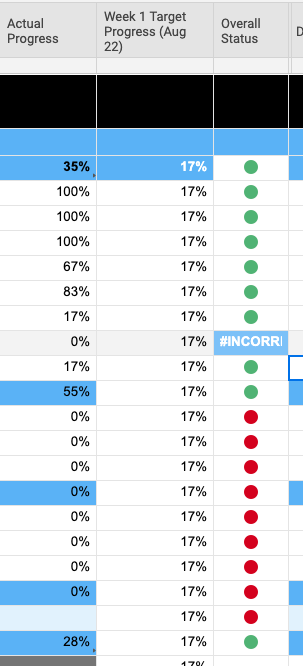# IF formula help to gray out a status

Hi Community...having issues with this formula working for the "Gray"Status.  The other criteria is working so can't figure out why I can't get gray status to work.

=IF([Actual Progress]10 >= (\$[Week 1 Target Progress (Aug 22)]10 * 0.8), "Green", IF(AND([Actual Progress]10 < (\$[Week 1 Target Progress (Aug 22)]10 * 0.8), [Actual Progress]10 >= (\$[Week 1 Target Progress (Aug 22)]10 * 0.6)), "Yellow", IF(AND([Actual Progress]10 < (\$[Week 1 Target Progress (Aug 22)]10 * 0.6), [Actual Progress]10 > 0, "Red", IF([Actual Progress]10 = 0, "Gray")))))• Your final AND is not closed. Try closing that and see if that helps.

• Hello

That is correct, as Paul mentioned above there appears to be a missing AND function, close parenthesis ), just before the value "Red". The below formula contains this correction.

=IF([Actual Progress]10 >= (\$[Week 1 Target Progress (Aug 22)]10 * 0.8), "Green", IF(AND([Actual Progress]10 < (\$[Week 1 Target Progress (Aug 22)]10 * 0.8), [Actual Progress]10 >= (\$[Week 1 Target Progress (Aug 22)]10 * 0.6)), "Yellow", IF(AND([Actual Progress]10 < (\$[Week 1 Target Progress (Aug 22)]10 * 0.6), [Actual Progress]10 > 0), "Red", IF([Actual Progress]10 = 0, "Gray")))))

Also, may want to try switching Gray to the first function. Depending on what values the formula produces it may be that order of operations isn't allowing Gray to appear. This would appear like this:

=IF([Actual Progress]10 = 0, "Gray", IF([Actual Progress]10 >= (\$[Week 1 Target Progress (Aug 22)]10 * 0.8), "Green", IF(AND([Actual Progress]10 < (\$[Week 1 Target Progress (Aug 22)]10 * 0.8), [Actual Progress]10 >= (\$[Week 1 Target Progress (Aug 22)]10 * 0.6)), "Yellow", IF(AND([Actual Progress]10 < (\$[Week 1 Target Progress (Aug 22)]10 * 0.6), [Actual Progress]10 > 0), "Red")))))

Have a wonderful day. Thank you for contacting Smartsheet Support.

Cheers,

Eric

Smartsheet Technical Support

• Eric,

I didn't really look at it this way until I read your post. The way the formula reads, all of the previous criteria sets have to be above 0 to be true which means the placement of the "Gray" shouldn't be an issue.

However... It got me to REALLY looking at the formula, and (correct me if I am wrong) I feel like we should be able to rewrite this without any AND statements. If you look at each AND, it is saying "if this is true and the previous criteria is false". If the formula has already progressed past the previous criteria, then by default it must be false.

.

This should work the same:

=IF([Actual Progress]@row >= \$[Week 1 Target Progress (Aug 22)]@row * 0.8, "Green", IF([Actual Progress]@row>= \$[Week 1 Target Progress (Aug 22)]@row* 0.6, "Yellow", IF([Actual Progress]@row> 0, "Red", "Gray")))

## Help Article Resources

Want to practice working with formulas directly in Smartsheet?

Check out the Formula Handbook template!